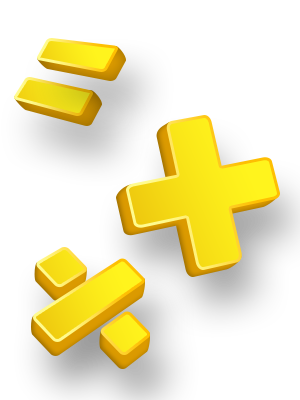A jump and run adventure game where you must find your way out by using logic, solving math puzzles and collecting coins.# Based on high-quality math standards### Learning Goals

The purpose of this amazing game is  to encourage kids to develop and practice their arithmetic skills including:

• Subtraction
• Multiplication
• Division

​At the beginning of the game, there is an option to select your challenges by category and by level of difficulty:

• Beginner
• Intermediate

Children from1st to 4th grade will be able to practice the skills they must know, based on the Common Core State Standards.

### Designed using Common Core Standards

Yash Math Adventure game was designed using the Common Core State Standards, who established consistent guidelines for what every student should know and be able to do in math from kindergarten through 12th grade.  The standards were drafted by experts and teachers from across the country.  They provide a way for teachers to measure student progress throughout the school year and ensure that students are on the pathway to success in their academic careers.

The following is a list of the Common Core Standards applied during the game:

• CCSS.MATH.CONTENT.1.OA.B.3  - Apply properties of operations as strategies to add and subtract.2 Examples: If 8 + 3 = 11 is known, then 3 + 8 = 11 is also known. (Commutative property of addition.) To add 2 + 6 + 4, the second two numbers can be added to make a ten, so 2 + 6 + 4 = 2 + 10 = 12. (Associative property of addition.)

• CCSS.MATH.CONTENT.1.OA.B.4 - Understand subtraction as an unknown-addend problem. For example, subtract 10 - 8 by finding the number that makes 10 when added to 8.

• CCSS.MATH.CONTENT.1.OA.C.6 - Add and subtract within 20, demonstrating fluency for addition and subtraction within 10. Use strategies such as counting on; making ten (e.g., 8 + 6 = 8 + 2 + 4 = 10 + 4 = 14); decomposing a number leading to a ten (e.g., 13 - 4 = 13 - 3 - 1 = 10 - 1 = 9); using the relationship between addition and subtraction (e.g., knowing that 8 + 4 = 12, one knows 12 - 8 = 4); and creating equivalent but easier or known sums (e.g., adding 6 + 7 by creating the known equivalent 6 + 6 + 1 = 12 + 1 = 13).

• CCSS.MATH.CONTENT.1.OA.D.8 - Determine the unknown whole number in an addition or subtraction equation relating three whole numbers. For example, determine the unknown number that makes the equation true in each of the equations 8 + ? = 11, 5 = _ - 3, 6 + 6 = _.

• CCSS.MATH.CONTENT.2.OA.B.2 - Fluently add and subtract within 20 using mental strategies.2 By end of Grade 2, know from memory all sums of two one-digit numbers.

• ​CCSS.MATH.CONTENT.2.OA.A.1 - Use addition and subtraction within 100 to solve one- and two-step word problems involving situations of adding to, taking from, putting together, taking apart, and comparing, with unknowns in all positions, e.g., by using drawings and equations with a symbol for the unknown number to represent the problem.

• CCSS.MATH.CONTENT.2.NBT.B.6 - Add up to four two-digit numbers using strategies based on place value and properties of operations.

• CCSS.MATH.CONTENT.3.OA.C.7 - Fluently multiply and divide within 100, using strategies such as the relationship between multiplication and division (e.g., knowing that 8 × 5 = 40, one knows 40 ÷ 5 = 8) or properties of operations. By the end of Grade 3, know from memory all products of two one-digit numbers.

• ​CCSS.MATH.CONTENT.3.OA.A.4 - Determine the unknown whole number in a multiplication or division equation relating three whole numbers. For example, determine the unknown number that makes the equation true in each of the equations 8 × ? = 48, 5 = _ ÷ 3, 6 × 6 = ?

• CCSS.MATH.CONTENT.3.OA.B.5 - Apply properties of operations as strategies to multiply and divide.2 Examples: If 6 × 4 = 24 is known, then 4 × 6 = 24 is also known. (Commutative property of multiplication.) 3 × 5 × 2 can be found by 3 × 5 = 15, then 15 × 2 = 30, or by 5 × 2 = 10, then 3 × 10 = 30. (Associative property of multiplication.) Knowing that 8 × 5 = 40 and 8 × 2 = 16, one can find 8 × 7 as 8 × (5 + 2) = (8 × 5) + (8 × 2) = 40 + 16 = 56. (Distributive property.)